### Math History

In the rest of this course, we'll focus on mathematics that relates to calculation, number, and equation solving. These are stories that connect ancient and modern algebra, algorithms, and number theory.

To begin digging into the number theory more, we'll need to discuss prime numbers. Again, attribution isn't really possible, but this time we know one real, ancient mathematician who did a lot of amazing mathematics.

Meet Eratosthenes.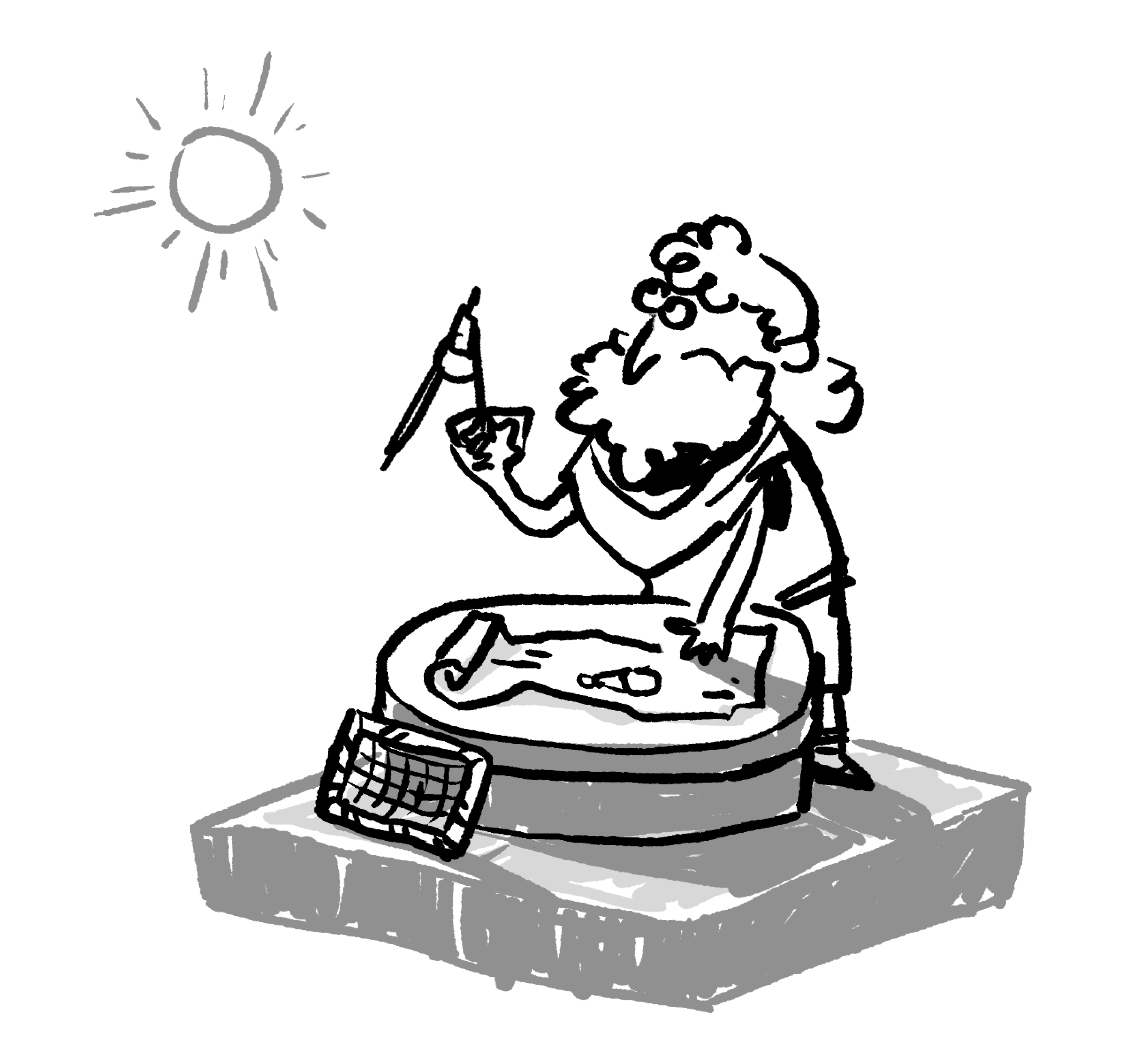# The Prime Sieve

Eratosthenes was a Greek mathematician (and astronomer, librarian, historian, poet, …) who lived around $200$ B.C. Sadly, almost everything he wrote himself has been lost forever, much of it likely consumed in the fire that destroyed the great library of Alexandria.

Although we don't have access to much of his written work, we do know some of Eratosthenes's accomplishments thanks to others who've referenced or quoted him.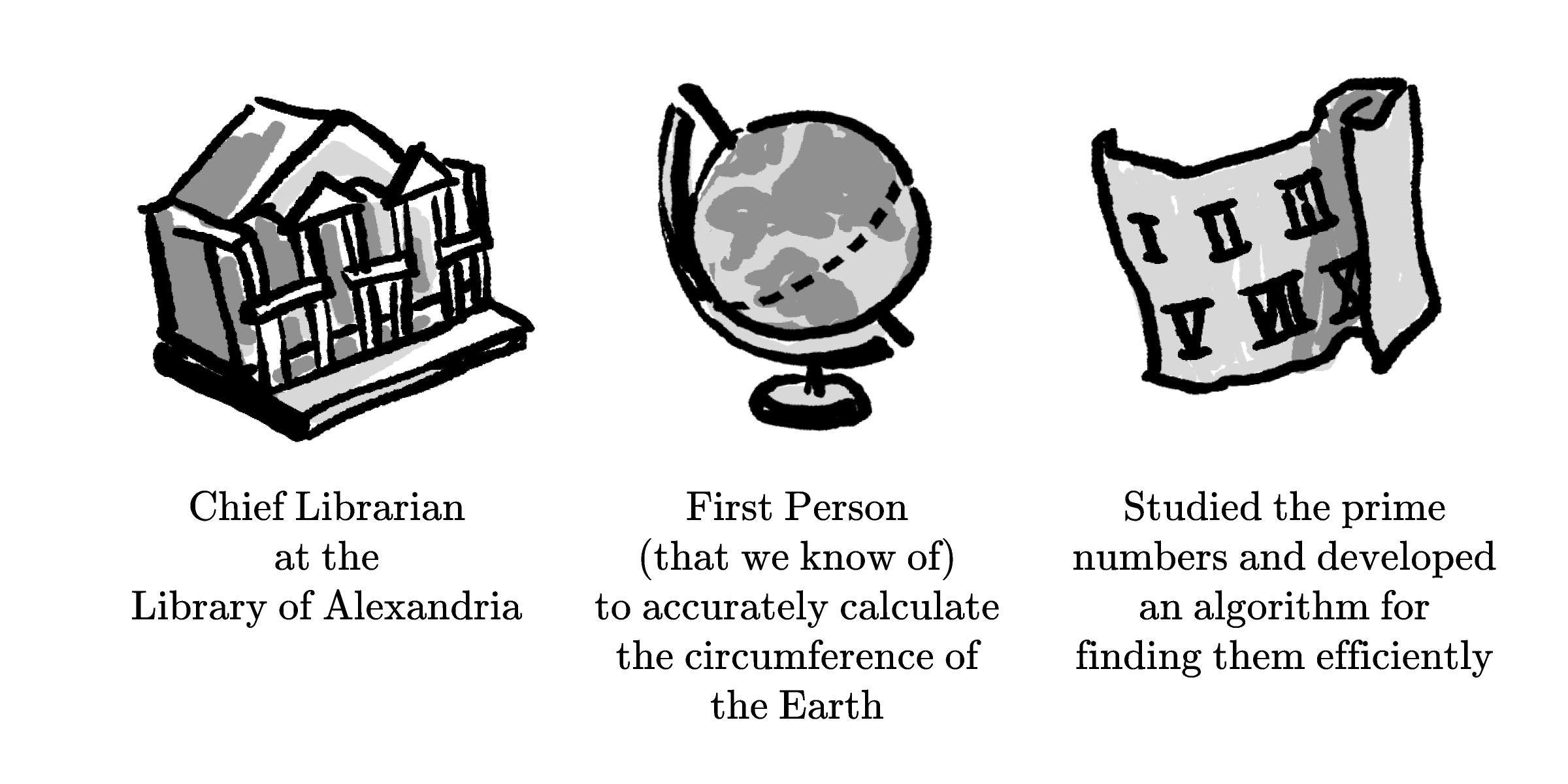# The Prime Sieve

Eratosthenes studied numbers, and one fundamental property of numbers is this: every positive integer greater than $1$ is either prime or composite. A prime number is divisible only by itself and $1,$ but composite numbers are divisible by at least one other number.

Prime numbers are like atoms (in the classical sense) — they are the building blocks out of which other numbers can be formed, but they themselves are indivisible. On the other hand, It's possible to think about composite numbers as if they are made out of primes: $12 = 2 \times 2 \times 3,$ for example.

With many problems, breaking down composite numbers into their prime pieces is quite helpful.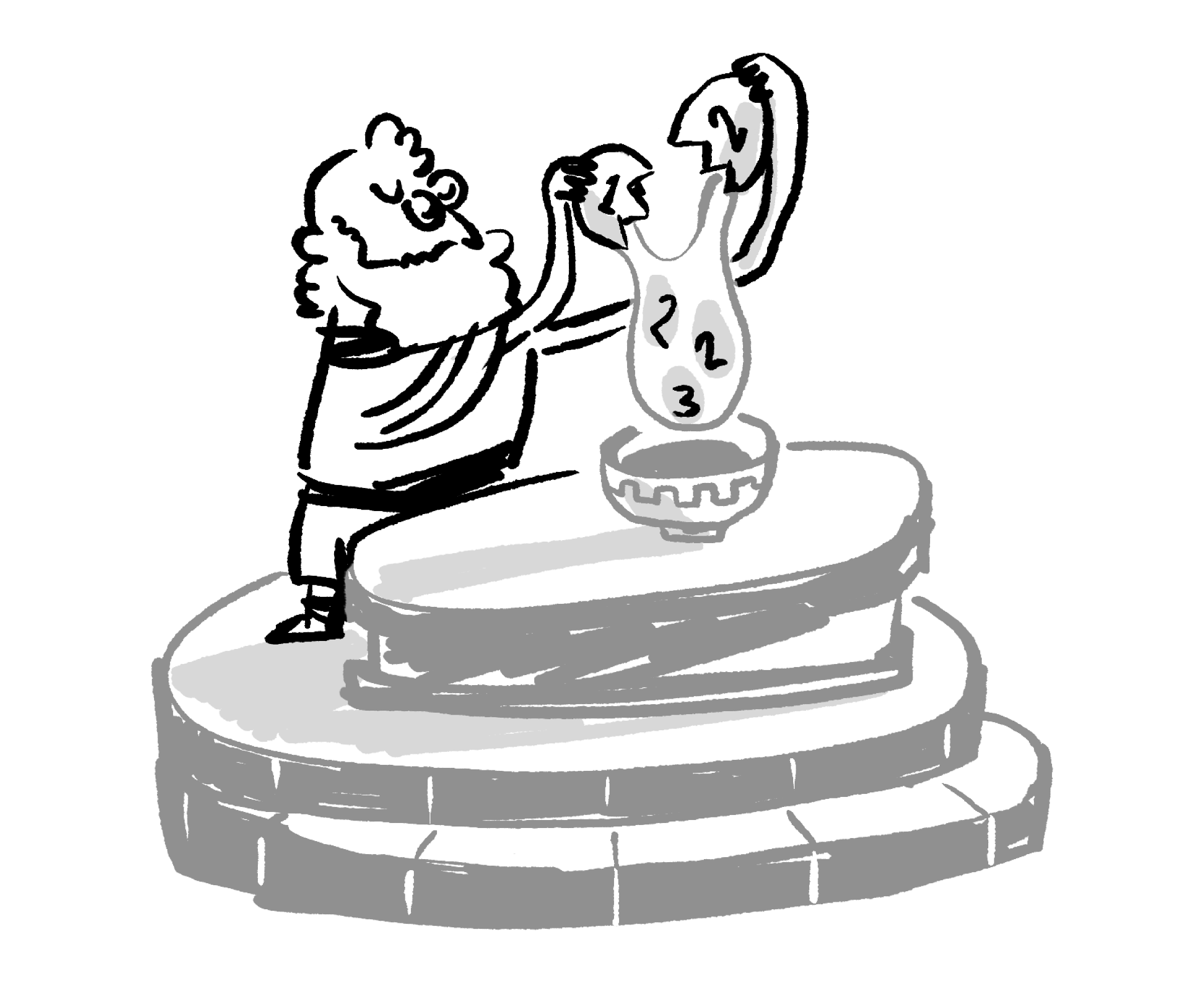# The Prime Sieve

$5, 7, 13, 23, \dots$

Prime numbers like these are the fundamental particles of the number universe, and much of number theory is built around the fact that every number is uniquely described as a product of its prime parts.

There's one big problem, though. We know next to nothing about prime numbers themselves (and as best we can tell, this bothered Eratosthenes quite a bit).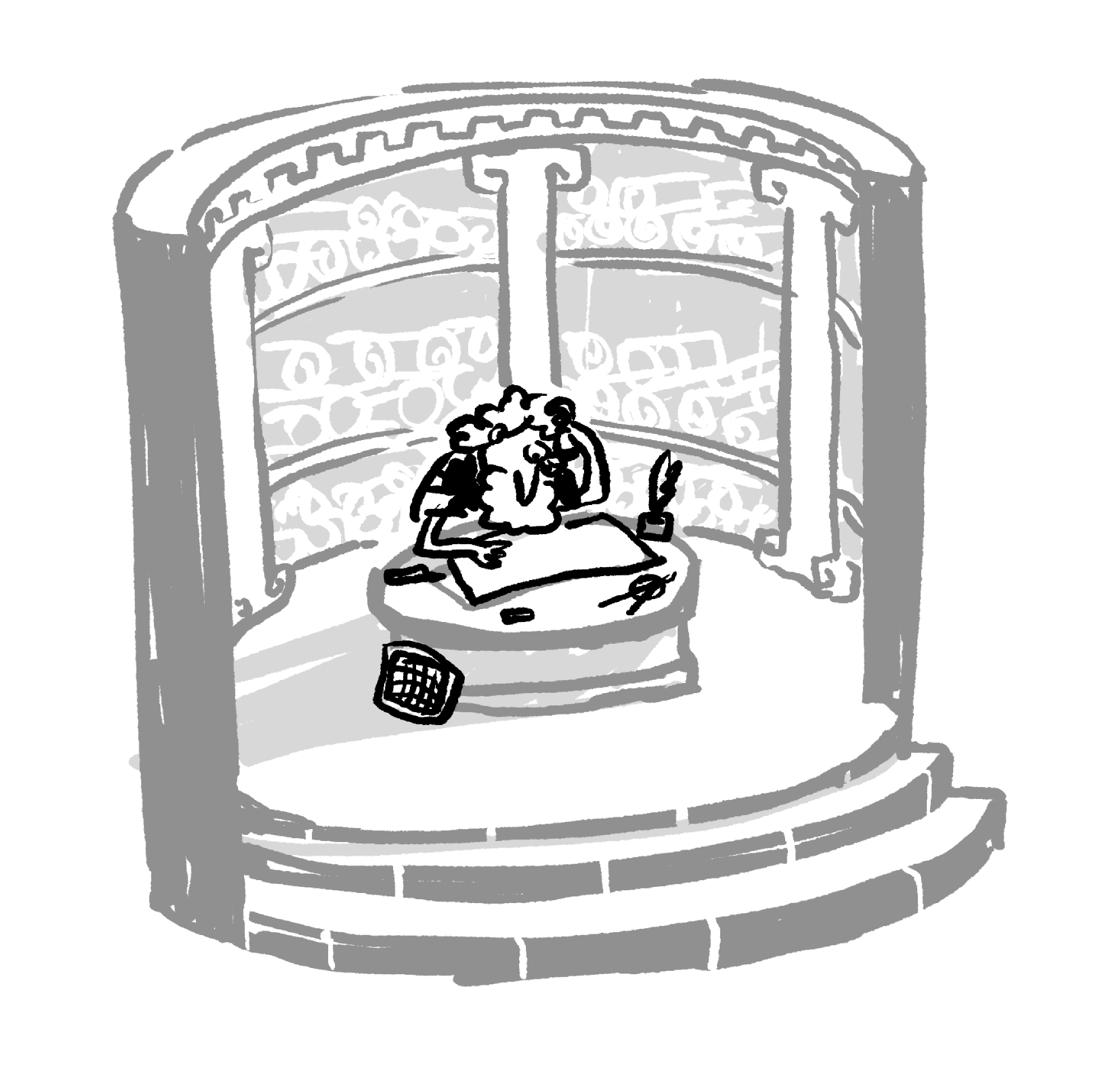# The Prime Sieve

One of the problems Eratosthenes set out to solve was a fundamental one: how do we even figure out which numbers are prime?

For small numbers, it's fairly quick to check: is $17$ prime? Let's see… it's not divisible by $2\dots$ it's not divisible by $3\dots$ it's not divisible by $4\dots$ and so on.

As numbers get larger, though, checking like this gets tedious very quickly. Eratosthenes set out to find a more efficient approach.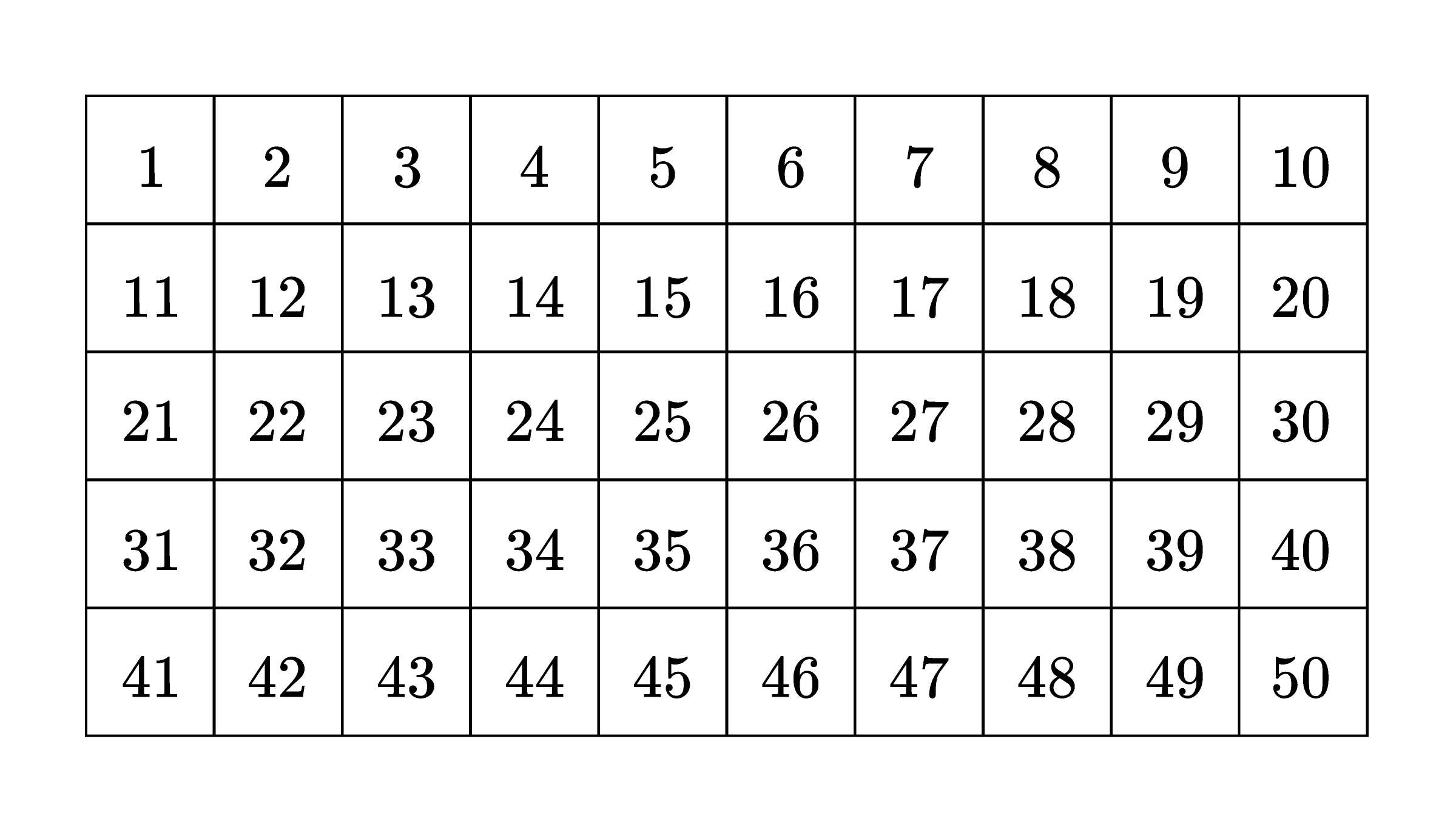How many of the numbers in this $1$$\text{-}$$50$ grid are multiples of $2?$

# The Prime Sieve

• $25$ numbers in this $1\text{-}50$ grid are multiples of $2.$
• $16$ numbers in this $1\text{-}50$ grid are multiples of $3.$

How many numbers from $1$ to $50$ are not multiples of $2$ or multiples of $3?$

# The Prime Sieve

The previous problems have hinted at a method that we can continue:

• Starting with $2,$ remove all multiples of $2$ from the list.
• Choose the next non-removed number and remove all of its multiples from the list. $\\[-1em]$

If we continue this procedure, which number is the last one that we'll end up using to remove a multiple of it (in addition to itself) from the list?# The Prime Sieve

The method we've just employed is known as the sieve of Eratosthenes. Like a sieve, it strains the numbers and leaves behind only the ones we want: the primes.

Eratosthenes's insight was as simple as it was profound. Finding primes is hard, but finding composite numbers is easy by comparison. Since every number is either one or the other, if we find all the composite numbers and remove them, then all the numbers left over will be prime.

Eratosthenes's sieve is a brilliant technique, and it was used for over a thousand years as the most efficient available technique for finding primes. But finding the primes might just raise more questions than it answers…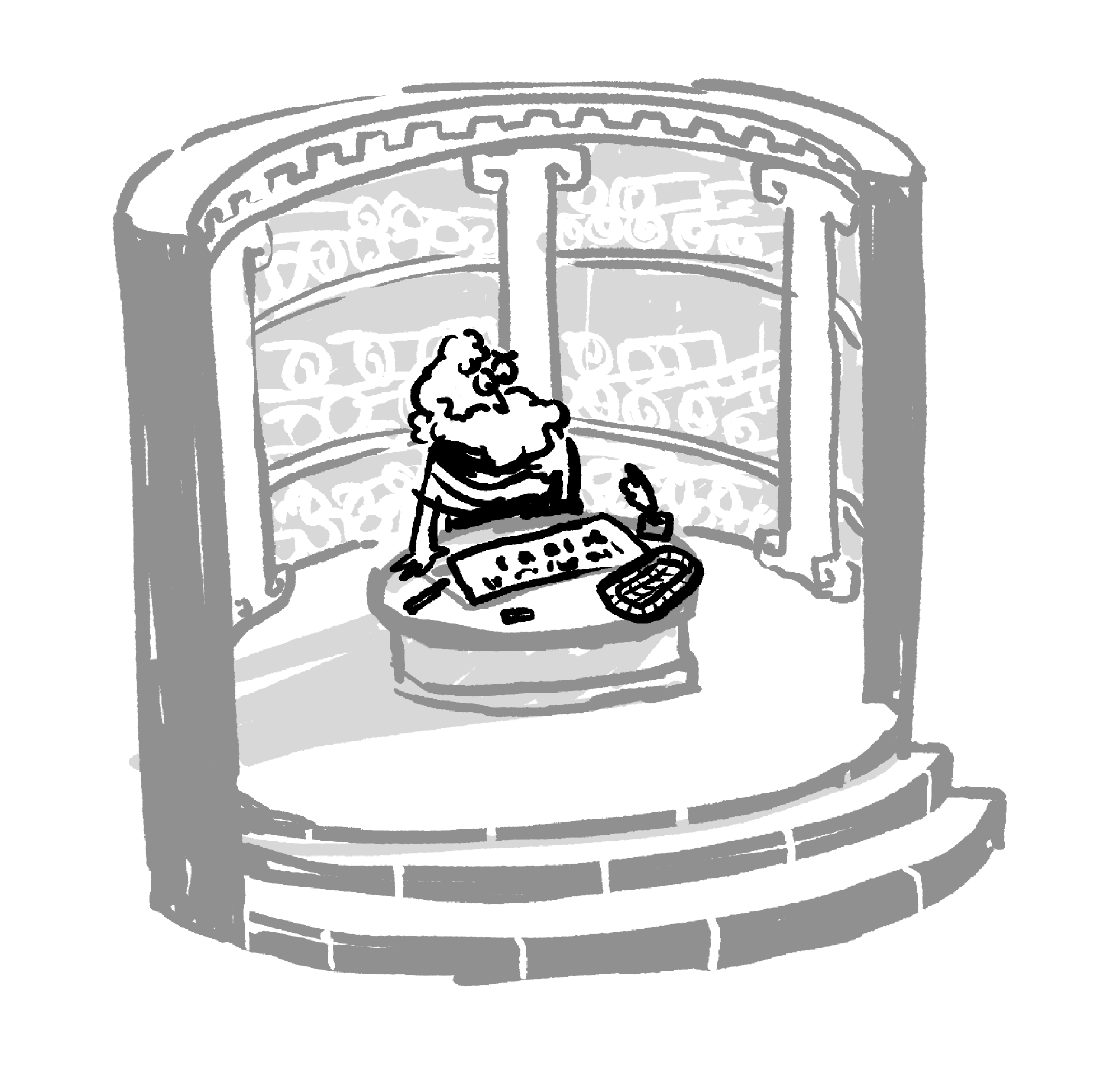# The Prime Sieve

Let's look at the first $25$ prime numbers, grouped by their leading digit:

$2, 3, 5, 7, \\ 11, 13, 17, 19, \\ 23, 29, \\ 31, 37, \\ 41, 43, 47,\\ 53, 59, \\ 61, 67, \\ 71, 73, 79, \\ 83, 89, \\ 97.$

What patterns are visible in the prime numbers here?

# The Prime Sieve

Select one or more

Although the primes don't have an obvious pattern, that hasn't stopped mathematicians through the centuries from looking for one.

Here's a simple formula that generates prime numbers: $x^2 - x + 5.$ Placing some values for $x$ into the formula can produce a prime number.

For example, placing $0$ for $x$ gives us $0^2 - 0 + 5 = 5,$ which is prime. Placing $3$ for $x$ gives us $11,$ another prime.

Which value of $x$ will produce a composite number instead of a prime?

# The Prime Sieve

Many people have attempted to improve Eratosthenes's approach by calculating prime number values directly. One example: the formula $x^2 + x + 41$ produces distinct primes for every integer value of $x$ from $0$ to $39.$ $($Without calculating anything, can you see why it definitely fails to make a prime when $x=41?)$

But despite some temporary successes like these, no formula or generating pattern has ever been found for the primes. And even now, over $2000$ years after Eratosthenes, we still don't have answers to some of the simplest questions:

• Are there infinitely many pairs of twin primes: two primes that are only two units apart, like $29$ and $31?$ No one knows.
• Why is it that, after a prime ending in $9,$ it seems almost twice as likely for the next prime to end in $1$ instead of $9?$ No one knows. (This might be a bias that only shows up for small primes.)
• Can every even number greater than $2$ be written as the sum of two primes? No one knows.

# The Prime Sieve

Like the other mathematicians we'll discuss, Eratosthenes made significant progress in mapping the landscape of numbers. What drove Eratosthenes to explore the primes, though? Was it curiosity about how much was still unknown, about how many questions still needed to be answered?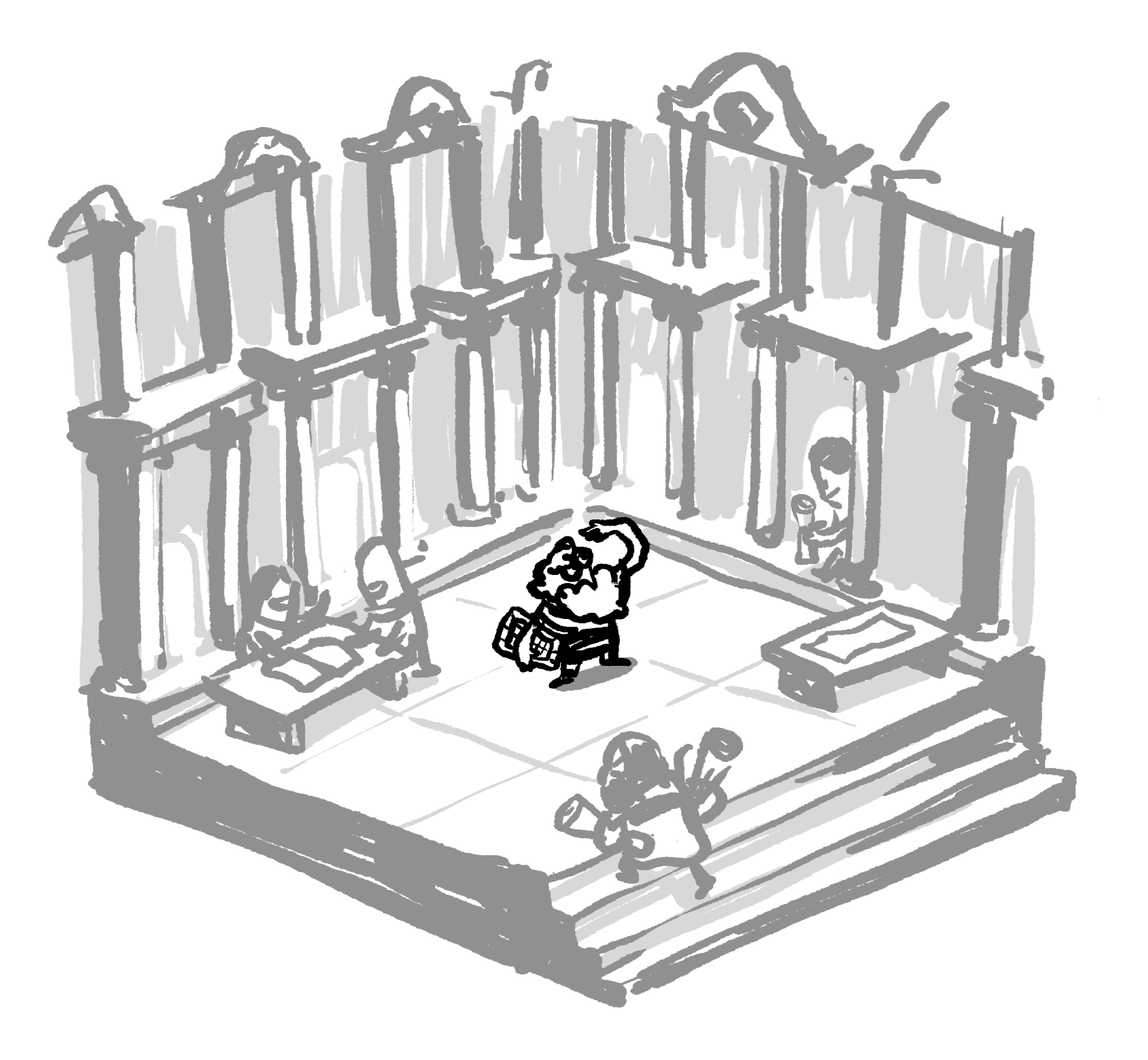Well, it often turns out that, in math, each new discovery and each new tool just helps us see that much farther into the wild tangle of that-which-we-still-do-not-know.

# The Prime Sieve

×